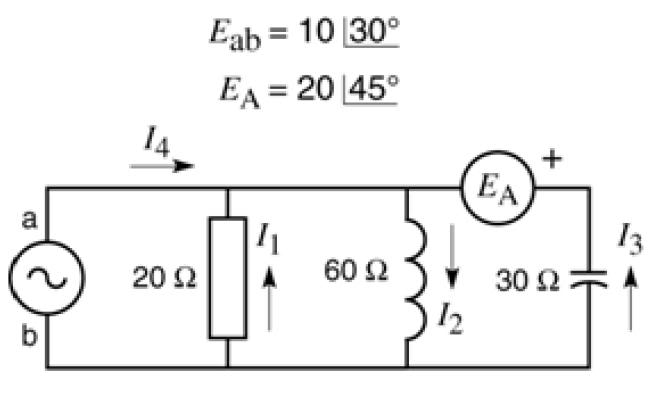# Kirchoff's Voltage Law in RLC circuit

## Homework Statement

I am required to write KVL circuit equations for the following circuitI don't need to finish solving for I1, I2, I3 and I4 at the moment. I just need a bit of help setting up the equations. Thanks.

## Homework Equations

Kirchoff's Voltage Law, which states that the sum of voltage drops in a loop must be equal to the sum of the voltage rises.

Ohm's Law, V = IR

## The Attempt at a Solution

There are a few things I am unsure of.

The first is that I don't know what the 30 degrees and the 45 degrees refers to beside the voltage sources. My first guess was that it means the phase that the alternating voltage source was up to at that particular moment in time, but I'm not sure if EA is even alternating, because it doesnt have the squigly line in the circle. In my attempted solution I guessed that both EA and Eab are alternating, and that the angle given refers to where in the phase it is at that point in time (with 0 degrees at the peak).

Another thing I'm unsure of is is the notation of Eab = 10. Does this mean the the potential at a is 10 higher than the potential at b? Or the other way around? I am unsure of the direction of the voltage. For my attempted solution I guessed that 'a' had the higher potential.

Anyway, here is my attempt at a solution:
Eab= 10 cos(30 degrees) = -20*I1

Eab= 10 cos (30 degrees) = 60*j*I2

Eab + EA= 10 cos (30 degrees) + 20 cos (45 degrees) = -30*-j*I3 = 30*j*I3

...
and from here I would be able to perform simultaneous equations to find the currents.

Any help would be greatly appreciated!!
Andrew

vela
Staff Emeritus
Homework Helper

## The Attempt at a Solution

There are a few things I am unsure of.

The first is that I don't know what the 30 degrees and the 45 degrees refers to beside the voltage sources. My first guess was that it means the phase that the alternating voltage source was up to at that particular moment in time, but I'm not sure if EA is even alternating, because it doesnt have the squigly line in the circle. In my attempted solution I guessed that both EA and Eab are alternating, and that the angle given refers to where in the phase it is at that point in time (with 0 degrees at the peak).
It's called Steinmetz notation. It just tells you the amplitude and relative phase of the signal. In your problem, $E_{ab}=10 \cos(\omega t+30^\circ)$ and $E_A = 20 \cos(\omega t+45^\circ)$.
Another thing I'm unsure of is is the notation of Eab = 10. Does this mean the the potential at a is 10 higher than the potential at b? Or the other way around? I am unsure of the direction of the voltage. For my attempted solution I guessed that 'a' had the higher potential.
"Higher" probably isn't the best word to use since it's an alternating source. The subscript "ab" usually means that you're measuring the voltage of point a relative to point b.

Both the things you said make sense. Thanks vela!

So, with this new understanding, I think the KVL equations are:

$E_{ab}=10 \cos(\omega t+30^\circ)$ = -20*I1

$E_{ab}=10 \cos(\omega t+30^\circ)$ = 60*j*I2

$E_{ab} + E_A= 10 \cos(\omega t+30^\circ) + 20 \cos(\omega t+45^\circ)$ = -30*-j*I3 = 30*j*I3

Which can then be solved to obtain the currents. Is this correct?

vela
Staff Emeritus
Homework Helper
Essentially, but you have a slight problem. The lefthand sides are real while the righthand sides are complex. You want to represent the voltages as a complex quantity and use that on the LHS of the equations.

Ok thanks. Representing the voltage as a complex quantity:

$$E_{ab}=10e^{\frac{\pi}{6}j}e^{j\omega t}=-20I_{1}$$

$$E_{ab}=10e^{\frac{\pi}{6}j}e^{j\omega t}=60jI_{2}$$

$$E_{ab} + E_A=10e^{\frac{\pi}{6}j}e^{j\omega t}+20e^{\frac{\pi}{4}j}e^{j\omega t}=-30jI_{3}$$

vela
Staff Emeritus